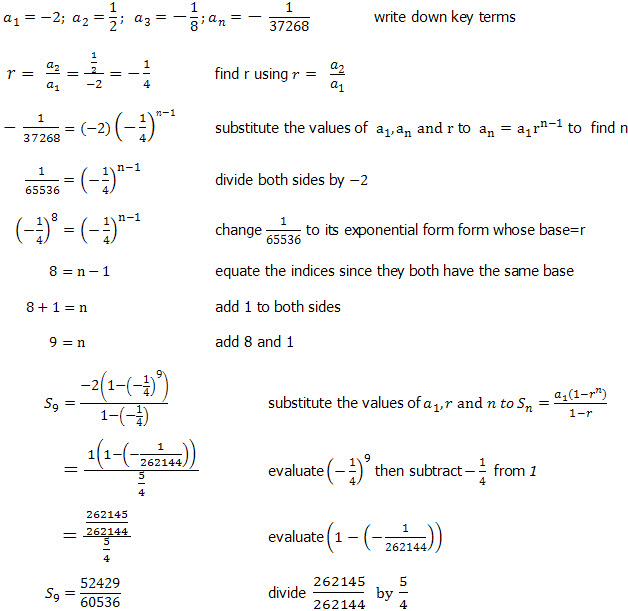×#### Thank you for registering.

One of our academic counsellors will contact you within 1 working day.

Click to Chat

1800-1023-196

+91-120-4616500

CART 0

• 0

MY CART (5)

Use Coupon: CART20 and get 20% off on all online Study Material

ITEM
DETAILS
MRP
DISCOUNT
FINAL PRICE
Total Price: Rs.

There are no items in this cart.
Continue Shopping```Geometric Progression

Geometric Progression as the name suggests is a kind of sequence in which the terms increase geometrically. This simply means that every next element is obtained by multiplying the previous element by a constant. This constant which is obtained by dividing the successive term by the preceding term is termed as the common ratio. The common ratio is generally denoted by ‘r’ and is equal to

r = a2/a1 = a3/a2 = ………. = an/an-1,

where r is the common ration and ai denotes the ith term.

The geometric progression may also be termed as the geometric sequence or GP in short.

Now, we aim at finding the sum of first n terms of a geometric progression, generally abbreviated as G.P.

Sn = a + ar + ar2 +…+ arn–1                              … (1)

Multiplying both sides by r, we get,

rSn = ar + ar2 +…+ arn–1 + arn                           … (2)

Subtracting (7) from (6), we have

Sn – rSn = a – arn

or, Sn = a(1–rn)/(1–r)                                        … (3)

From equation (3), it follows that the sum of n terms of a G.P.

Sn = a(1–rn)/(1–r)

Hence, the sum of first n terms of a G.P. is given by the formula

Sn = a (1–rn)/ (1–r)

Watch this Video for more reference

We now wish to consider the case when n tends to infinity.

The case of n tending to infinity will depend on the value of |r|. we consider two cases:

If the value of |r| is greater than 1 then as n tends to infinity, rn will also become infinite. Hence, in this case, S∞ will also tend to infinity.

Similarly, if we consider the case when the value of |r| < 1, then as n tends to infinity, rn tends to zero. In this case, S∞ = a/1–r for |r| < 1.

Result: We now discuss the cases when any real number is added, subtracted, multiplied or divided to each term of the geometric series.

1. If all the terms of a G.P. are multiplied or divided by a constant number, the    resultant sequence is also a G.P.

Suppose a1, a2, a3, ……, an are in G.P.

then ka1, ka2, ka3, ……, kan and

a1/k, a2/k, ... ... ... an/k will also be in G.P.

where k ∈R and k ≠ 0.

2. The multiplication or division of two geometric progressions is also a geometric progression.

Suppose a1, a2, a3, ……, an and b1, b2, b3, ……, bn are two G.P.

then a1b1, a2b2, a3b3, ……, anbn

and a1/b1, a2/b2, ... ... ..., an/bn will also be in G.P.

3. Reversing the order of a G.P.’s also results a G.P.

Suppose a1, a2, a3, ……, an are in G.P.

then an, an–1, an–2, ……, a3, a2, a1 will also be in G.P.

4. Taking the inverse of a G.P. also results a G.P.

Suppose a1, a2, a3, ……, an are in G.P.

then 1/a1, 1/a2, 1/a3 ……, 1/an will also be in G.P.

Remark: Generally, questions are also asked in exam on finding the three numbers of G.P. satisfying certain condition. Though it is not mandatory, but if the numbers are assumed in a particular manner, it becomes easy to solve questions. We suggest students to assume the geometric variables in the following manner:

If we need to assume three numbers in G.P. then a/b, a, ab    here common ratio is b

Four number in G.P.   a/b3, a/b, ab, ab3   here common ratio is b2

Five numbers in G.P.    a/b2, a/b, a, ab, ab2  here common ratio is b

Illustration: Find the sum of the geometric series -2, ½, -1/8, …., -1/37268

Solution: Writing the terms of the geometric progressionSome key points to be noted:

If each term of a G.P. is multiplied or divided by a fixed non-zero constant then the new sequence is also a G.P. with the same common ratio.

If each term of a G.P. (with common ratio r) is raised to the power k, then the resulting sequence is also a G.P. with common ratio rk.

If there are two G.P.s with different ratios say

a1, a2, a3, ……, is a G.P. with common ratio r
b1, b2, b3, …… is the second G.P. with common ratio r’ respectively then the sequence a1b1, a2b2, a3b3, …… is also a G.P. with common ratio rr’.

We have stated above the process of assuming three, four or five terms in a G.P. In general, if we need to assume (2k + 1) terms in a G.P., they can be assumed as a/rk, a/rk–1, …, a, ar, …, ark.

If 2k terms need to be assumed then the terms of the G.P. can be assumed as a/r2k–1, a/r2k–3, ... ... ... a/r, ar, ……, ar2k–1.

If a1, a2, ……, an are in G.P., then a1an = a2an–1 = a3an–2 = ……

If a1, a2, a3, …… is a G.P. (each a1 > 0), then loga1, loga2, loga3 …… is an A.P. The converse is also true.

askIITians offers comprehensive study material which contains all the important topics of IIT JEE Mathematics. The most frequently asked questions of the JEE have also been covered in detail. It is important to master this topic in order to remain competitive in the JEE.

Related Resources

Look into the Previous Year Papers with Solutions to get a hint of the kinds of questions asked in the exam.

You can get the knowledge of Useful Books of Mathematics here

```### Course Features

• 731 Video Lectures
• Revision Notes
• Previous Year Papers
• Mind Map
• Study Planner
• NCERT Solutions
• Discussion Forum
• Test paper with Video Solution